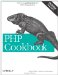# Recipe 4.14. Finding the Largest or Smallest Valued Element in an Array

#### 4.14.1. Problem

You have an array of elements, and you want to find the largest or smallest valued element. For example, you want to find the appropriate scale when creating a histogram.

#### 4.14.2. Solution

To find the largest element, use max( ):

`\$largest = max(\$array);`

To find the smallest element, use min( ):

`\$smallest = min(\$array);`

#### 4.14.3. Discussion

Normally, max( ) returns the larger of two elements, but if you pass it an array, it searches the entire array instead. Unfortunately, there's no way to find the index of the largest element using max( ). To do that, you must sort the array in reverse order to put the largest element in position 0:

`arsort(\$array);`

Now the value of the largest element is \$array.

If you don't want to disturb the order of the original array, make a copy and sort the copy:

`\$copy = \$array; arsort(\$copy);`

The same concept applies to min( ) but uses asort( ) instead of arsort( ).

Recipe 4.16 for sorting an array; documentation on max( ) at http://www.php.net/max, min( ) at http://www.php.net/min, arsort( ) at http://www.php.net/arsort, and asort( ) at http://www.php.net/asort.PHP Cookbook: Solutions and Examples for PHP Programmers
ISBN: 0596101015
EAN: 2147483647
Year: 2006
Pages: 445

Similar book on Amazon